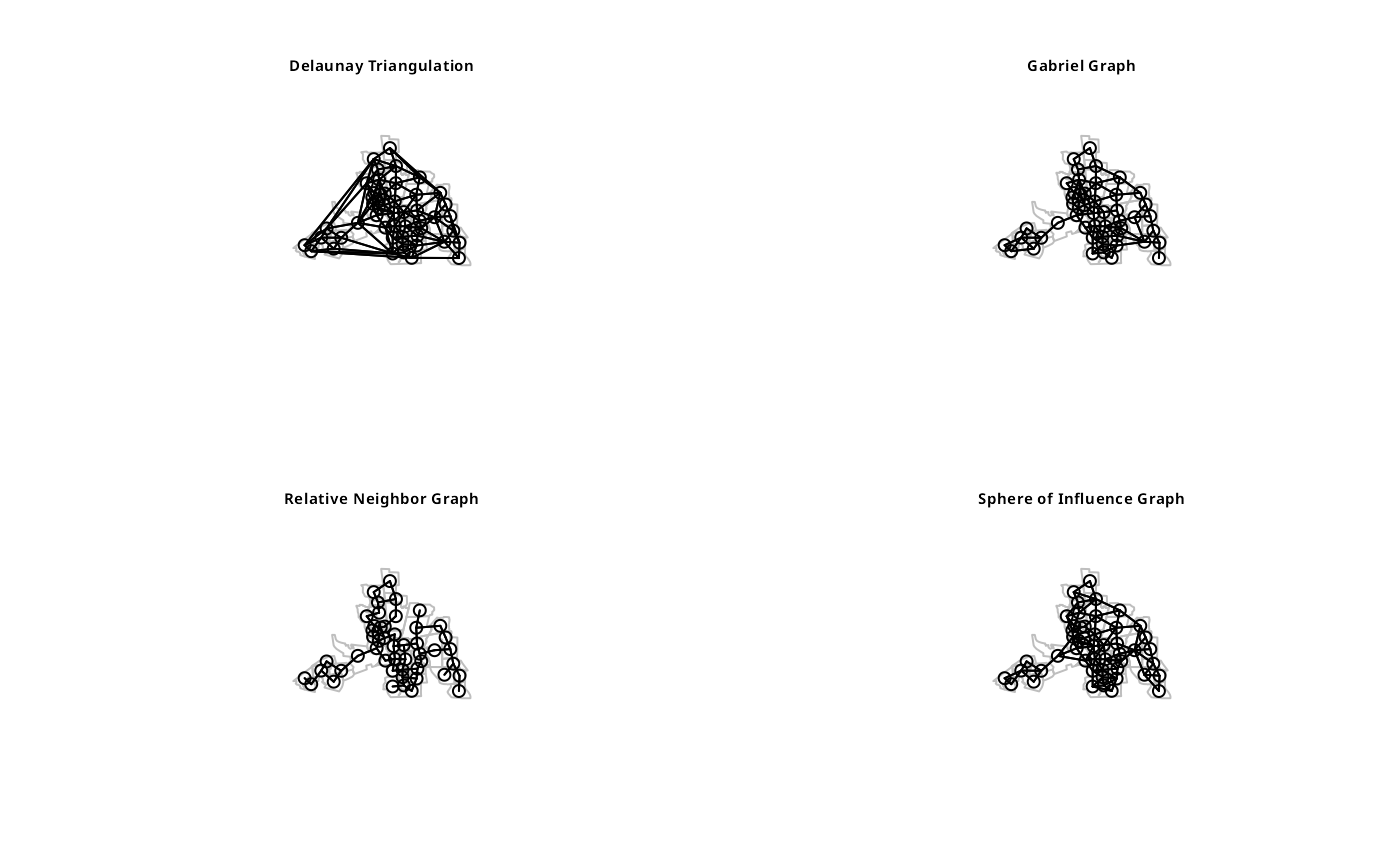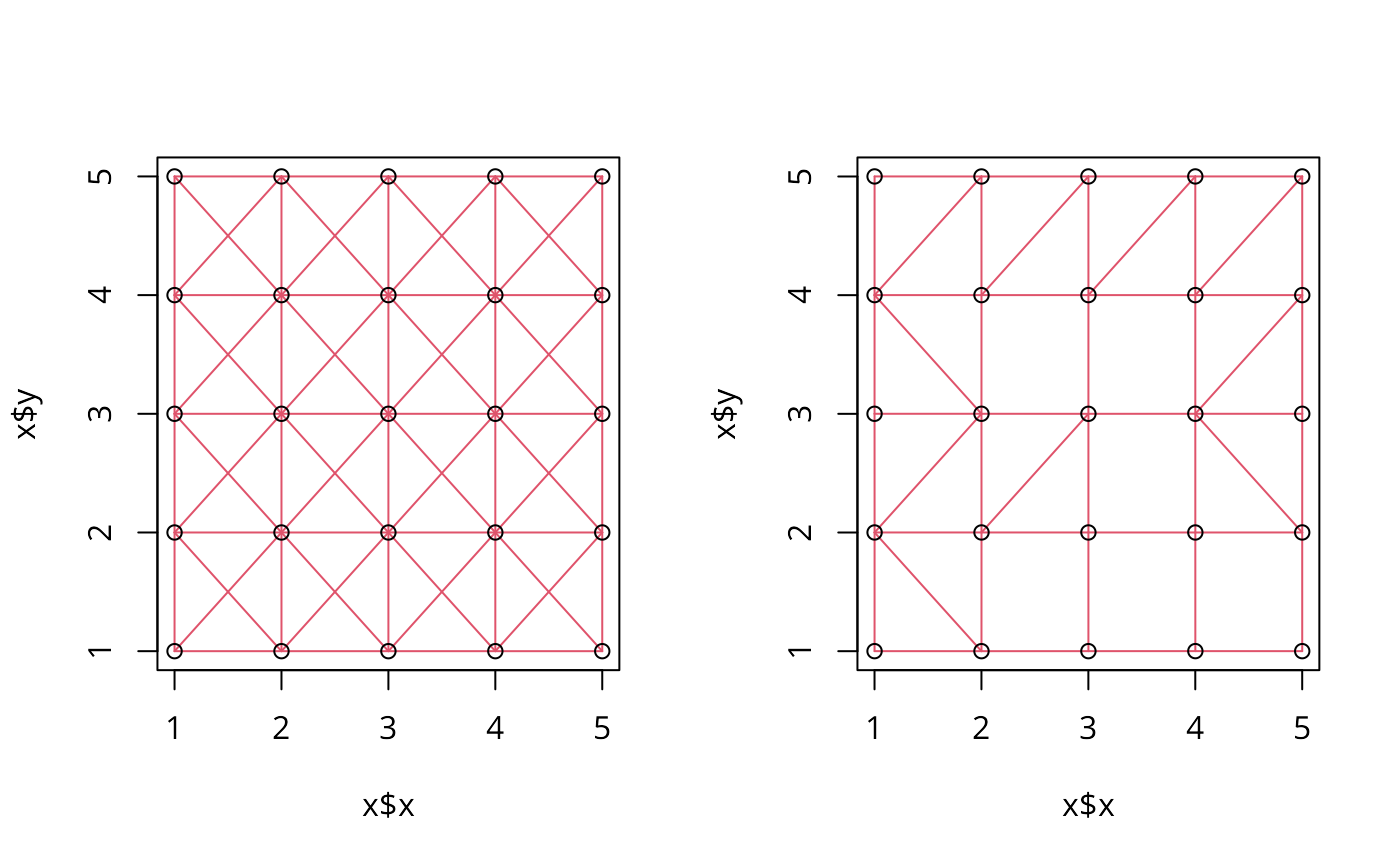Functions return a graph object containing a list with the vertex coordinates and the to and from indices defining the edges. Some/all of these functions assume that the coordinates are not exactly regularly spaced. The helper function graph2nb converts a graph object into a neighbour list. The plot functions plot the graph objects.

gabrielneigh(coords, nnmult=3)
relativeneigh(coords, nnmult=3)
<!-- %beta.skel(coords,beta) -->
graph2nb(gob, row.names=NULL,sym=FALSE)
# S3 method for Gabriel
# S3 method for relative
plot(x, show.points=FALSE, add=FALSE, linecol=par(col),...)

## Arguments

coords

matrix of region point coordinates or SpatialPoints object or sfc points object

nnmult

scaling factor for memory allocation, default 3; if higher values are required, the function will exit with an error; example below thanks to Dan Putler

tri.nb

a neighbor list created from tri2nb

number of line segments making a quarter circle buffer, see the nQuadSegs argument in geos_unary

gob

a graph object created from any of the graph funtions

row.names

character vector of region ids to be added to the neighbours list as attribute region.id, default seq(1, nrow(x))

sym

a logical argument indicating whether or not neighbors should be symetric (if i->j then j->i)

x

object to be plotted

show.points

linecol

edge plotting colour

...

further graphical parameters as in par(..)

## Details

The graph functions produce graphs on a 2d point set that are all subgraphs of the Delaunay triangulation. The relative neighbor graph is defined by the relation, x and y are neighbors if

$$d(x,y) \le min(max(d(x,z),d(y,z))| z \in S)$$

where d() is the distance, S is the set of points and z is an arbitrary point in S. The Gabriel graph is a subgraph of the delaunay triangulation and has the relative neighbor graph as a sub-graph. The relative neighbor graph is defined by the relation x and y are Gabriel neighbors if

$$d(x,y) \le min((d(x,z)^2 + d(y,z)^2)^{1/2} |z \in S)$$

where x,y,z and S are as before. The sphere of influence graph is defined for a finite point set S, let $$r_x$$ be the distance from point x to its nearest neighbor in S, and $$C_x$$ is the circle centered on x. Then x and y are SOI neigbors iff $$C_x$$ and $$C_y$$ intersect in at least 2 places. From 2016-05-31, Computational Geometry in C code replaced by calls to functions in dbscan and sf; with a large quadsegs= argument, the behaviour of the function is the same, otherwise buffer intersections only closely approximate the original function.

See card for details of “nb” objects.

## Value

A list of class Graph with the following elements

np

number of input points

from

array of origin ids

to

array of destination ids

nedges

number of edges in graph

x

input x coordinates

y

input y coordinates

The helper functions return an nb object with a list of integer vectors containing neighbour region number ids.

## Author

Nicholas Lewin-Koh nikko@hailmail.net

knearneigh, dnearneigh, knn2nb, card

## Examples

columbus <- st_read(system.file("shapes/columbus.shp", package="spData"), quiet=TRUE)
sf_obj <- st_centroid(st_geometry(columbus), of_largest_polygon)
sp_obj <- as(sf_obj, "Spatial")
coords <- st_coordinates(sf_obj)
suppressMessages(col.tri.nb <- tri2nb(coords))
col.gab.nb <- graph2nb(gabrielneigh(coords), sym=TRUE)
col.rel.nb <- graph2nb(relativeneigh(coords), sym=TRUE)
par(mfrow=c(2,2))
plot(st_geometry(columbus), border="grey")
title(main="Delaunay Triangulation", cex.main=0.6)
plot(st_geometry(columbus), border="grey")
title(main="Gabriel Graph", cex.main=0.6)
plot(st_geometry(columbus), border="grey")
title(main="Relative Neighbor Graph", cex.main=0.6)
plot(st_geometry(columbus), border="grey")
if (require("dbscan", quietly=TRUE)) {
col.soi.nb <- graph2nb(soi.graph(col.tri.nb,coords), sym=TRUE)
title(main="Sphere of Influence Graph", cex.main=0.6)
}par(mfrow=c(1,1))
col.tri.nb_sf <- tri2nb(sf_obj)
all.equal(col.tri.nb, col.tri.nb_sf, check.attributes=FALSE)
#>  TRUE
col.tri.nb_sp <- tri2nb(sp_obj)
all.equal(col.tri.nb, col.tri.nb_sp, check.attributes=FALSE)
#>  TRUE
if (require("dbscan", quietly=TRUE)) {
col.soi.nb_sf <- graph2nb(soi.graph(col.tri.nb, sf_obj), sym=TRUE)
all.equal(col.soi.nb, col.soi.nb_sf, check.attributes=FALSE)
col.soi.nb_sp <- graph2nb(soi.graph(col.tri.nb, sp_obj), sym=TRUE)
all.equal(col.soi.nb, col.soi.nb_sp, check.attributes=FALSE)
}
#>  TRUE
col.gab.nb_sp <- graph2nb(gabrielneigh(sp_obj), sym=TRUE)
all.equal(col.gab.nb, col.gab.nb_sp, check.attributes=FALSE)
#>  TRUE
col.gab.nb_sf <- graph2nb(gabrielneigh(sf_obj), sym=TRUE)
all.equal(col.gab.nb, col.gab.nb_sf, check.attributes=FALSE)
#>  TRUE
col.rel.nb_sp <- graph2nb(relativeneigh(sp_obj), sym=TRUE)
all.equal(col.rel.nb, col.rel.nb_sp, check.attributes=FALSE)
#>  TRUE
col.rel.nb_sf <- graph2nb(relativeneigh(sf_obj), sym=TRUE)
all.equal(col.rel.nb, col.rel.nb_sf, check.attributes=FALSE)
#>  TRUE
dx <- rep(0.25*0:4,5)
dy <- c(rep(0,5),rep(0.25,5),rep(0.5,5), rep(0.75,5),rep(1,5))
m <- cbind(c(dx, dx, 3+dx, 3+dx), c(dy, 3+dy, dy, 3+dy))
cat(try(res <- gabrielneigh(m), silent=TRUE), "\n")
#> Error in gabrielneigh(m) : number of neighbours overrun - increase nnmult
#>
res <- gabrielneigh(m, nnmult=4)
summary(graph2nb(res))
#> Neighbour list object:
#> Number of regions: 100
#> Number of nonzero links: 342
#> Percentage nonzero weights: 3.42
#> Average number of links: 3.42
#> 1 region with no links:
#> 100
#> Non-symmetric neighbours list
#>
#>  0  1  2  3  4  5
#>  1  8 10 18 55  8
#> 8 least connected regions:
#> 46 47 48 49 96 97 98 99 with 1 link
#> 8 most connected regions:
#> 10 15 20 25 30 35 40 45 with 5 links
grd <- as.matrix(expand.grid(x=1:5, y=1:5)) #gridded data
r2 <- gabrielneigh(grd)
set.seed(1)
grd1 <- as.matrix(expand.grid(x=1:5, y=1:5)) + matrix(runif(50, .0001, .0006), nrow=25)
r3 <- gabrielneigh(grd1)
opar <- par(mfrow=c(1,2))
plot(r2, show=TRUE, linecol=2)
plot(r3, show=TRUE, linecol=2)par(opar)
class(coords)
#>  "spec_tbl_df" "tbl_df"      "tbl"         "data.frame"
graph2nb(gabrielneigh(coords))
#> Neighbour list object:
#> Number of regions: 100
#> Number of nonzero links: 179
#> Percentage nonzero weights: 1.79
#> Average number of links: 1.79
#> 23 regions with no links:
#> 22 39 43 53 58 61 66 70 71 73 76 78 81 88 90 93 94 95 96 97 98 99 100
#> Non-symmetric neighbours list
graph2nb(relativeneigh(coords))
#> Neighbour list object:
#> Number of regions: 100
#> Number of nonzero links: 117
#> Percentage nonzero weights: 1.17
#> Average number of links: 1.17
#> 31 regions with no links:
#> 22 29 33 39 41 42 43 44 53 58 61 64 65 66 70 71 73 75 76 78 81 88 90 93
#> 94 95 96 97 98 99 100
#> Non-symmetric neighbours list